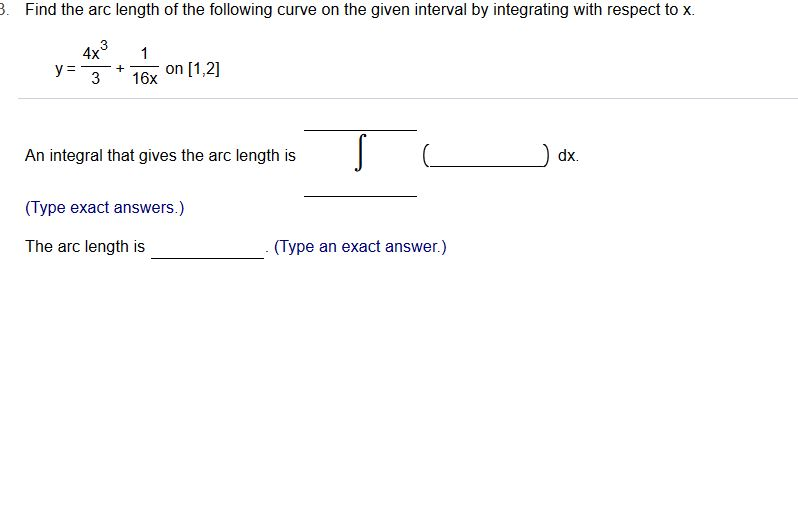# .Find the arc length of the following curve on the given interval by integrating with respect to x.4x1y316x on [1,2]An integral that gives the arc length is(Type exact answers.)The arc length isdx.(Type an exact answer.)

Question
7 viewshelp_outlineImage Transcriptionclose. Find the arc length of the following curve on the given interval by integrating with respect to x. 4x1 y316x on [1,2] An integral that gives the arc length is (Type exact answers.) The arc length is dx. (Type an exact answer.) fullscreen
check_circle

Step 1

Given an equation of a curve  as shown below, we need to find the length of the arc  from [1,2].

Step 2

For any curve f(x) on [a,b] the length of the arc is given by the following formula.

Step 3

Let us calculate the derivative of given curve with respect to x. After calculating t...

### Want to see the full answer?

See Solution

#### Want to see this answer and more?

Solutions are written by subject experts who are available 24/7. Questions are typically answered within 1 hour.*

See Solution
*Response times may vary by subject and question.
Tagged in

### Integration